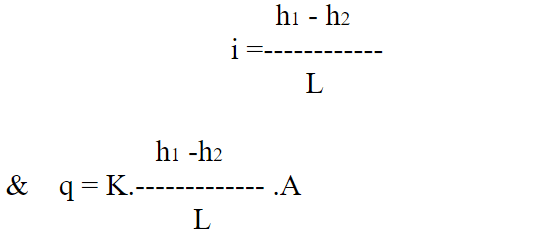# Permeability of Soil – Darcy’s law – Limitation, Coefficient of Permeability, Importance of Study of Seepage Analysis

4th April 2018

## Permeability of Soil

Permeability is defined as the property of a porous material which permits the passage or seepage of water (or other fluids)through its interconnecting voids.

A material having continuous voids is called permeable. Gravels are highly permeable, while stiff clay is the least permeable.

### 4 Importance Of Study Of Seepage Analysis

The study of seepage of water through soils is important for the following engineering problems:

1. Determination of rate of settlement of a saturated compressible soil layer
2. Calculation of seepage through the body of earth dams, and stability of slopes.
3. Calculation of uplift pressure under the hydraulic structure and their safety against piping
4. Groundwater flow towards wells and drainage of soils.

### DARCY’S LAW

The law of flow of water through the soil was first studied by Darcy. This law states that the rate of flow or discharge per unit time (q) is proportional to the hydraulic gradient (i).

This law only for laminar flow conditions in a saturated soil mass. If q is the discharge per unit time through a soil mass of cross-sectional area ‘A’ under hydraulic gradient ‘i’.

Then, q ∞ i.A or, q= K.i.A

Where,
K is a constant, known as the coefficient of permeability.
If a soil sample of length ‘L’ and cross-sectional area ‘A’ (measured perpendicular to the direction of flow) is subjected to a differential head of water (h1-h2), then

### Limitation Of DARCY’S LAW

Darcy’s law is valid only for laminar flow condition in the soil mass.

### Coefficient Of Permeability

The coefficient of permeability is defined as the average velocity of flow that will occur through the total cross-sectional area of the soil mass under a unit hydraulic gradient. It is denoted by ‘K’ Its unit is the same as the unit of velocities such as Cm/sec or m/sec or m/hr, etc.